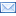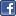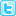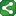## Tuesday, March 3, 2009

### Calculating group totals and the counts within each group

Sample 25217: Calculating group totals and the counts within each group

This example uses the SUM() function to sum the AMOUNT column, creating a new column named GRPTOTAL with a COMMA10. format. The COUNT() function counts the number of occurrences of STATE within each group.

The GROUP BY clause collapses multiple rows for each group into one row per group, containing STATE, GRPTOTAL and the COUNT.

data one; input state \$ amount;
cards;
CA 7000
CA 6500
CA 5800
NC 4800
NC 3640
SC 3520
VA 4490
VA 8700
VA 2850
VA 1111

proc sql;
create table two as select state ,sum(amount) as grptotal format=comma10. , count(*) as count from one group by state;
quit

proc print data=two noobs;
run;ShareThis